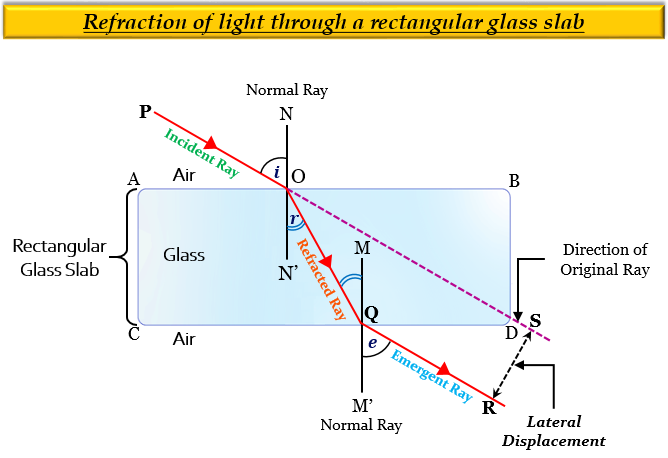# (a) Draw a labelled ray diagram to show the path of a ray of light incident obliquely on one face of a glass slab. (b) Calculate the refractive index of the material of a glass slab. Given that the speed of light through the glass slab is 2 x 108 m/s and in air is 3 x 108 m/s.(c) Calculate the focal length of a lens, if its power is ŌĆō 2┬Ę5 D.

(a) Labelled ray diagram to show the path of a ray of light incident obliquely on one face of a glass slab is given below:(b) Given:

The speed of light through the glass slab = $2\times {10^8m/s}$

The speed of light in air = $3\times {10^8m/s}$

To find: Refractive index of the material of a glass slab, $n_{ga}$.

Solution:

We know that the formula of refractive index $(n)$ is given as-

$Refractive\ index\ of\ a\ medium=\frac {Speed\ of\ light\ in\ vacuum/air}{Speed\ of\ light\ in\ medium}$

Here,

$n_{ga}=\frac {Speed\ of\ light\ in\ air}{Speed\ of\ light\ in\ glass}$

Putting the given values in the expression we get-

$n_{ga}=\frac {3\times {10^8m/s}}{2\times {10^8m/s}}$

$n_{ga}=\frac {3}{2}$

$n_{ga}=1.5$

Thus, the refractive index of the material of a glass slab, $n_{ga}$ is 1.5.

(c) Given:

Power of the lens, $P$ = $-$2.5 D

To find: Focal length, $f$.

Solution:

We know that formula for power of lens is given as-

$P=\frac {1}{f}$

Putting the value of $f$ in the formula, we get-

$-2.5=\frac {1}{f}$

$f=-\frac {1}{2.5}$

$f=-\frac {10}{25}$

$f=-0.4m$

Thus, the focal length, $f$ of the lens is -4m.

Updated on: 10-Oct-2022

93 Views